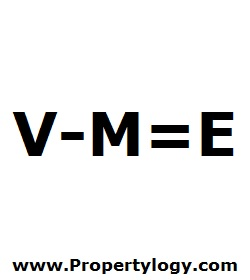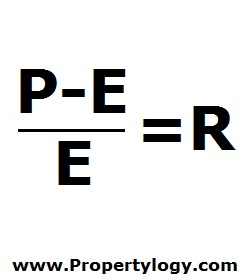Formula - Return on Equity | Propertylogy
Don't Miss

# Formula – Return on Equity

By on June 11, 2018

In order to find the return on equity of an asset, the amount of equity has to be tabulated first.V – M = E

V – Current market value

M – Mortgage balance owing

E – Equity

Equity on a property refers to the difference of the market value of a property and the amount outstanding of a mortgage debt.

With equity calculated using the formula above, we can now use it to calculate return on equity using the below equation.(P – E)/E = R

P – Proceeds from sale

E – Equity

R = Return on equity

Unlike return on investment which works out the returns based on investment capital, return on equity works out the returns based on equity.

Expressed as a percentage, this is used as a measurement of how profitable an investment is.

You May Also Like...##### How to burn more calories in the office

Send this to a friend